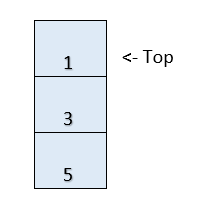×

Search anything:

# Reverse first K elements of Queue using Stack

#### Algorithms queue StackTo reverse the first K elements of a queue, we can use an auxiliary stack. We push the first k elements in the stack and pop() them out so and add them at the end of the queue. Popping the elements out of the queue reverses them.

We can do this in linear time O(N) with O(K) space.

To bring the remaining n-k elements to their correct positions, we simply need to remove them from the front and add them at the end of the queue. After this operation the first K elements of the queue will be reversed. The complete algorithm is discussed below with an example and implementation.

## Algorithm

The steps to Reverse first K elements of Queue using Stack are:

1. Create a stack.
2. Dequeue the first K elements of the queue and push them into the stack in order.
3. Pop the elements out of the stack and Enqueue (add) them into the queue.
4. Now, remove (Dequeue) the remaining N-K elements from the queue and insert (Enqueue) them at the end of the queue.

Key ideas:

• Queue is FIFO (First In First Out)
• Stack is LIFO (Last In First Out)
• If you Dequeue and Enqueue in order for K times, then the last K elements of Queue becomes first K elements. As Queue is FIFO, the first out element becomes "last in" for this case.
• If we do (Dequeue from Queue, Push into Stack) for K times and then )Pop from Stack, Enqueue for Queue) for K times, then elements are reversed. This is because First In element from Queue is First Out and is inserted first in Stack. From Stack, the last in element comes out first so the last element of queue is inserted first now.

## Implementation

Following is the implementation of our approach in C++:

``````void reverseK(queue q, int k)
{
if(k <= 0)
return;

if(q.size() == 0 || q.size() == 1)
return;
int n = q.size();
stack<int> s;
for(int i=0;i<k;i++)        //for reversing first k elts
{
s.push(q.front());
q.pop();
}
while(s.empty() == false)      //adding the reversed elts back into the Q
q.push(s.pop());

for(int i=0;i<n-k;i++)
{
q.push(q.front());
q.pop();
}
}
``````

Following is the implementation of our approach in Java:

``````public static void reverseK(Queue<Integer> q, int k)
{
if(k <= 0)
return;
if(q.size() == 0 || q.size() == 1)
return;

int n = q.size();
Stack<Integer> s = new Stack<>();
for(int i=0;i<k;i++)        //for reversing first k elts
{
s.push(q.peek());
q.poll();
}
while(s.isEmpty() == false)      //adding the reversed elts back into the Q

for(int i=0;i<n-k;i++)
{
q.poll();
}
}
``````

## Example

Consider a queue Q containing 7 elements:
Q:

5 3 1 2 6 10 9

and let K = 3. We need to reverse the first K elements of the queue.

• According to the algorithm discussed above, we first create a stack.
• We then push the first k elements in the stack, which leads to the following states of the queue and the stack:

Q:

2 6 10 9

Stack:• Pop all the elements out of the stack now, and add them to the queue.

Q:

2 6 10 9 1 3 5
• Now, we transfer the remaining n-k (7 - 3 = 4) elements from the front of the queue to the end.
6 10 9 1 3 5 2
10 9 1 3 5 2 6
9 1 3 5 2 6 10

The required queue

1 3 5 2 6 10 9

# Time & Space Complexity

• Time complexity: O(N)

Each element is removed from the queue exactly once and added back to the queue exactly once. As the add() and remove() functions take O(1) time, the time complexity is upper bounded by O(N).

• Space Complexity: O(K)

Only the first K elements of the queue need to go into the stack. Hence the space complexity is O(K).

With this article at OPENGENUS, you must have the complete idea of reversing the first K elements of a Queue.